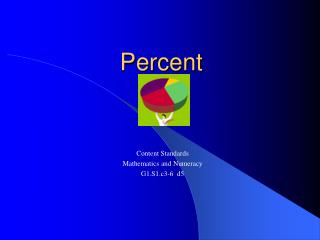DownloadDownload PresentationPercent

# Percent

Télécharger la présentation## Percent

- - - - - - - - - - - - - - - - - - - - - - - - - - - E N D - - - - - - - - - - - - - - - - - - - - - - - - - - -
##### Presentation Transcript

1. Percent Content Standards Mathematics and Numeracy G1.S1.c3-6 d5

2. Definition • Percent can be defined as “of one hundred.” of 100

3. 20 parts out of 100 or 20 hundredths. 20 % Means

4. 20 100 1 5 Percents Have Equivalents in Decimals and Fractions Fraction Simplified Percent Decimal Fraction 20% .20 = = =

5. How to Find the Percent of a Whole Number • The first thing to remember is “of” means multiply in mathematics. of x =

6. How to Find the Percent of a Whole Number • Step 1 - When you see a percent problem you know when you read “of” in the problem you multiply. x 25% of 200

7. How to Find the Percent of a Whole Number • Step 2 – Change your percent to a decimal and then move it two places to the left. . . 25% x 200

8. How to Find the Percent of a Whole Number • Step 3 – Multiply just like a regular decimal multiplication problem. 200 x . 25 1000 +400 5000

9. How to Find the Percent of a Whole Number • Step 4 – Place the decimal point 2 places to the left in your answer. 200 x . 25 1000 +400 . 5000

10. What is the percent of this number? . 20 % of 820 . x 820 x . 20 000 +1640 . 16400

11. What is the percent of this number? . x 40 % of 725 . 1 2 725 x . 40 000 +2900 . 29000

12. What is the percent of this number? . x 60 % of 692 . 5 1 692 x . 60 000 +4152 . 21520